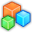# Microsoft Small BasicProgram Listing: MBL863
' Known Issue - Wide Line Rotates Not from Center

GraphicsWindow.Title = "Known Issue - Wide Line Rotates Not from Center"
GW_DrawGrid()
x = 100
y = 80
width = 120
height = 240
GraphicsWindow.PenWidth = width
GraphicsWindow.BrushColor = "#66000000"
x1 = 0
y1 = 0
x2 = 0
y2 = height
line = Shapes.AddLine(x1, y1, x2, y2)
Shapes.Move(line, x + width / 2, y)
GraphicsWindow.PenWidth = 0
GraphicsWindow.BrushColor = "#660099FF"
GraphicsWindow.BrushColor = "#6600FF00"
GraphicsWindow.FillRectangle(x, y, width, height)
Shapes.Move(rect, x, y)
While "True"
For angle = 5 To 360 Step 5
Shapes.Rotate(line, angle)
Shapes.Rotate(rect, angle)
If Math.Remainder(angle, 90) = 0 Then
Program.Delay(1000)
Else
Program.Delay(200)
EndIf
EndFor
EndWhile

Sub GW_DrawGrid
' GraphicsWindow | draw grid
_gw = GraphicsWindow.Width
_gh = GraphicsWindow.Height
_c100 = "#66000000"
_c10 = "#33000000"
For _x = 0 To _gw Step 10
If Math.Remainder(_x / 10, 10) = 0 Then
GraphicsWindow.PenColor = _c100
Else
GraphicsWindow.PenColor = _c10
EndIf
GraphicsWindow.DrawLine(_x, 0, _x, _gh)
EndFor
For _y = 0 To _gh Step 10
If Math.Remainder(_y / 10, 10) = 0 Then
GraphicsWindow.PenColor = _c100
Else
GraphicsWindow.PenColor = _c10
EndIf
GraphicsWindow.DrawLine(0, _y, _gw, _y)
EndFor
EndSub